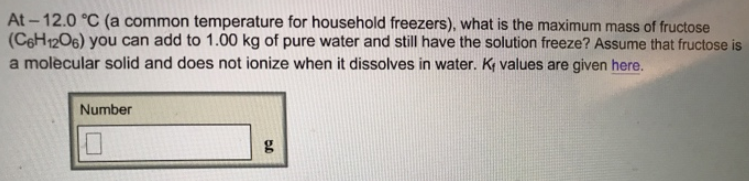Chemistry Practice Problems Freezing Point Depression Practice Problems Solution: At -12.0°C (a common temperature for household fre...

# Solution: At -12.0°C (a common temperature for household freezers), what is the maximum mass of fructose (C6H12O6) you can add to 1.00 kg of pure water and still have the solution freeze? Assume that fructose is a molecular solid and does not ionize when it dissolves in water. Kf values are given here.

###### Problem

At -12.0°C (a common temperature for household freezers), what is the maximum mass of fructose (C6H12O6) you can add to 1.00 kg of pure water and still have the solution freeze? Assume that fructose is a molecular solid and does not ionize when it dissolves in water. Kf values are given here.View Complete Written Solution

Freezing Point Depression

Freezing Point Depression

#### Q. Calculate the freezing point and the boiling point of a 0.050 m MgCl  2 solution using the observed van’t Hoff factors in Table 10‑6.

Solved • Mon Oct 29 2018 10:12:58 GMT-0400 (EDT)

Freezing Point Depression

#### Q. lf a 0.770 m aqueous solution freezes at -3.30°C, what is the van't Hoff factor, i, of the solute? Kf values can be found here.

Solved • Tue Oct 02 2018 11:08:47 GMT-0400 (EDT)

Freezing Point Depression

#### Q. The following experimental data was collected during a freezing point depression study. Use this data to determine Kf for BHT (butylated hydroxytoluen...

Solved • Tue Oct 02 2018 11:06:43 GMT-0400 (EDT)

Freezing Point Depression

#### Q. Choose the aqueous solution below with the  highest freezing point. These are all solutions of nonvolatile solutes and you should assume ideal van't H...

Solved • Mon Oct 01 2018 15:41:14 GMT-0400 (EDT)